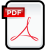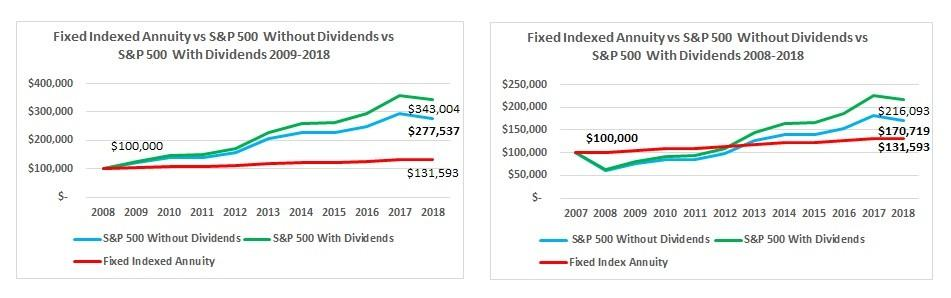# Understanding Fixed Indexed Annuities – 11/01/19Money Matters – Skloff Financial Group Question of the Month – November 1, 2019

Q:  We read the Understanding Annuities article and are interested in fixed indexed annuities (FIAs).  Can you please explain FIAs?

The Problem – Fixed Indexed Annuities (FIAs) Can be Confusing

Fixed index annuities (FIAs), also known as an equity indexed annuities (EIAs), can be complex and contain a litany of rules and restrictions.

The Solution – Understanding Fixed Indexed Annuities (FIAs)

Let’s start with defining a fixed indexed annuity.  FIAs are fixed annuities that earn interest based on price changes (excluding dividends) in an index, such as the S&P 500.  Once interest is credited to your annuity for an index term, those earnings usually are locked in and declines in the index do not reduce values.

Understanding How You Earn Interest and How Your Interest Rate May Differ from Your Index Return

Insurance companies use different formulas to calculate the interest added to your annuity, as described below.

Fixed Indexed Formulas.  The annual point-to-point formula credits interest based on the change in the index calculated using two dates, one year apart.  The multi-year point-to-point formula credits interest based on the change in the index calculated using two dates, more than one year apart.  The monthly point-to-point formula credits interest based on the change in the index calculated for each month during the index term. Monthly changes are limited to the “cap rate” for positive changes, but not negative changes. At the end of the index term, all monthly changes (positive and negative) are added. If the result is positive, interest is added to the annuity, if negative or zero, no interest is added.

Indexed Interest Rate.  Your indexed interest rate is impacted by additional factors.  The participation rate dictates how much of the increase in the index is used to calculate index-linked interest. A participation rate usually is for a set period. The period can be from one year to the entire term.  The cap rate is the maximum rate of interest the annuity will earn during the index term. Some annuities guarantee that the cap rate will never be lower (higher) than a set minimum (maximum). The spread rate is a set percentage the insurer subtracts from any change in the index. Translation: the participation rate, cap rate and spread rate could be decreased each year.

Numbers Speak Louder Than Words.  Let’s look at two examples.  In the first example an investor purchases a \$100,000 fixed annuity based on an annual point-to-point price change in the S&P 500, with an 80% participation rate and 4% rate cap, at the beginning of 2009.  At the end of 2018 (a total of 10 years) the annuity is worth \$131,593.  Had the investor invested in a vehicle that generated a return equal to the S&P 500 without dividends, the value at the end of 2018 would have been \$277,537.  Had the investor invested in a vehicle that generated a return equal to the S&P 500 with dividends, the value at the end of 2018 would have been \$343,004.  See the chart below.

In the second example an investor purchases a \$100,000 fixed annuity with the same terms at the beginning of 2008, right before the S&P 500 returned -37%; the worst year in the stock market since 1931 – during the Great Depression.  At the end of 2018 (a total of 11 years) the annuity is worth \$131,593.  Had the investor invested in a vehicle that generated a return equal to the S&P 500 without dividends, the value at the end of 2018 would have been \$170,719.  Had the investor invested in a vehicle that generated a return equal to the S&P 500 with dividends, the value at the end of 2018 would have been \$216,093.  See the chart below.

## Click to EnlargeIndex Interest Rates Versus Index Return in Extreme Years.  In the first example, the 1st year return for the S&P 500 with dividends was 26.5%, while the FIA credit was 4% interest.  In the second example, the 1st year return for the S&P 500 with dividends was -37%, while the FIA credit was 0% interest (with no loss in value). Despite the FIA’s protection in negative years, the S&P 500 significantly outperformed.

Action Steps – Understand the Capabilities and Limitations of Fixed Indexed Annuities

Before purchasing a FIA evaluate frequency at which the insurance company can change the indexes available, participation rates, cap rates and spread rates.  Work closely with an experienced financial professional to understand all the capabilities and limitations of FIAs.

Aaron Skloff, Accredited Investment Fiduciary (AIF), Chartered Financial Analyst (CFA), Master of Business Administration (MBA) is CEO of Skloff Financial Group, a Registered Investment Advisory firm specializing in financial planning, investment management and benefits for small to middle sized companies. He can be contacted at www.skloff.com or 908-464-3060.